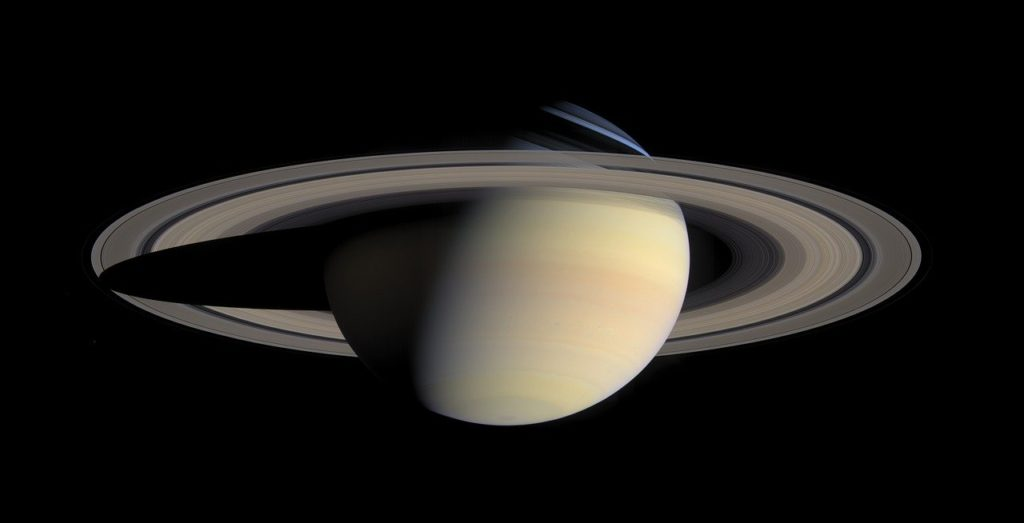# Saturn facts – orbit, physical overview, rings, etc.

Saturn is the secondlargest planet in our solar system. It is the sixth planet from the sun and it is probably the most easily recognizable planet after Earth. Let’s learn some interesting facts about Saturn, the “Lord of the rings”.

## Saturn facts

#### Orbit

• Saturn orbits at a distance of 886 million miles (average) from the Sun. 886 million miles is approximately equal to 9.5 Astronomical Units (AU). 1 Astronomical Unit is the average distance of Earth from the Sun. In other words, Saturn is 9.5 times as far as Earth is from the Sun.

886 million miles = 1.4 billion kilometers

• Saturn takes 10,759 Earth days to complete one revolution around the Sun. However Saturn’s rotation speed is quite high. It completes one rotation around its axis in just 10.7 hours.

1 Saturn year = 29.5 Earth years
1 Saturn day = 0.45 Earth days

#### Physical overview

• Saturn is a gas giant. Its atmosphere is mainly made up of Hydrogen and Helium. There is a dense core at the center which is relatively very small as compared to the total size. The core is made up of metals like Iron and Nickel.
• There is an absence of true surface at Saturn.
• The average density of Saturn is very less, even less than water.

Saturn’s density = 687 kg / m3
Density of Water = 997 kg / m3
Earth’s density = 5,514 kg / m3

Saturn is the least dense planet in our solar system.

• Saturn is 95 times heavier than Earth.

Mass of Earth = 5.97 × 10^24 kg
Mass of Saturn = 568.34 × 10^24 kg

• The winds in the equatorial region of Saturn can reach a speed of 500 m/s.

500 m/s = 1800 km/hr = 1118.5 miles/hr.

• Saturn’s rotational axis is tilted at an angle of 26.73 degrees with respect to its orbital axis. Our Earth’s rotational axis is tilted at an angle of 23.5 degrees with respect to its orbital axis.
• There is a huge difference between Saturn’s equatorial and polar radii. Saturn is in fact, the flattest planet of our solar system.

Equatorial radius = 60,268 km
Polar radius = 54,364 km
Mean radius = 58,232 km

• Saturn is 9 times wider than Earth.

Saturn’s mean radius = 58,232 km
Earth’s mean radius = 6,371 km

• Saturn’s magnetic moment is 578 times stronger than Earth’s magnetic moment.Saturn

#### Rings

Everyone knows that Saturn is the planet with rings around it. However, it is not the only planet with rings. Jupiter, Uranus, and Neptune also have rings but their rings are very faint.

Saturn has a complex ring system. Its rings are made up of chunks of ice, rocks, and dust. The rings are named as A, B, C, D, E, F, and G in the order of their discovery.

#### Natural satellites

• Saturn is the planet with the most number of natural satellites. There are 82 moons of Saturn. Jupiter has 3 fewer moons, 79.
• Titan is the largest moon of Saturn. It is also the second-largest moon in our Solar system. The largest moon of our solar system is the largest moon of Jupiter, Ganymede.
• Titan is the only place in our solar system besides Earth where stable liquids exist in the form of lakes, seas, and rivers. These liquid bodies are made up of carbon compounds like methane and ethane.
• Titan is even bigger in diameter than Mercury.

#### Additional facts about Saturn

• Saturn is one of the five planets visible with the naked eye. Mercury, Venus, Mars, Jupiter, and Saturn are visible from Earth with the naked eye.
• Saturn was named after the Roman God of wealth and agriculture.
• 4 spacecraft have visited Saturn, NASA’s Pioneer 11, Voyager 1 and Voyager 2 and Cassini-Huygens.

#### Quick question

How many known moons does Mars has ?

#### Answer to quick question

Mars has 2 known moons, Phobos and Deimos.